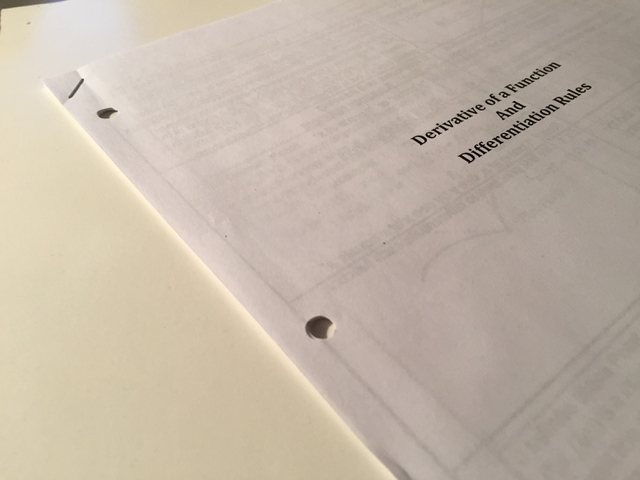## TLE '16 Contest 7 P1 - Math Helper

View as PDF

Points: 5 (partial)
Time limit: 2.0s
Memory limit: 256M

Author:
Problem typesA stapled package on simple derivatives - with lots of practice!

While the CS nerd is preparing for various computing competitions, the girl approaches him! She gives him some calculus homework and asks him to do it for her help her complete it. Not wanting to waste a valuable opportunity, the CS nerd agrees to help.

The girl takes out a large stapled package of paper with many simple calculus problems. Her task is to take the derivative of each equation on the sheet.

Each equation is in the form y = ax^b, whereandare given integers. The derivative of this equation (more accurately, the derivative ofwith respect to) is y' = abx^(b-1). The derivative should also be as simplified as possible. In particular, all these rules must be satisfied:

• 0x^n should be simplified to 0
• kx^0 should be simplified to k
• 1x^n whereshould be simplified to x^n
• -1x^n whereshould be simplified to -x^n
• kx^1 whereshould be simplified to kx
• 1x^1 should be simplified to x
• -1x^1 should be simplified to -x
• kx^-n whereshould not be in fraction form (i.e. it should not be k/(x^n))

A given equation may be in its simplified form as described above.

There areproblems on the worksheet. Can you help the CS nerd finish this tedious homework?

#### Input Specification

The first line will contain a single integer,, the number of problems.

The nextlines of input each contain an equation in the form y = ax^b, which could be in a more simplified form, but not completely simplified. It is guaranteed thatand.

#### Output Specification

For each of theequations, output the derivative on a separate line.

#### Sample Input

7
y = x^5
y = 2x^2
y = 100
y = -3x^-2
y = 5x
y = -x^10
y = 0x^0

#### Sample Output

y' = 5x^4
y' = 4x
y' = 0
y' = 6x^-3
y' = 5
y' = -10x^9
y' = 0